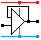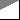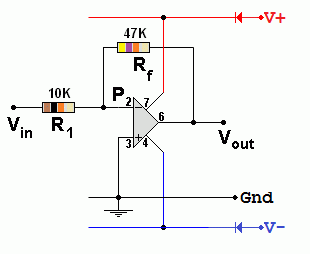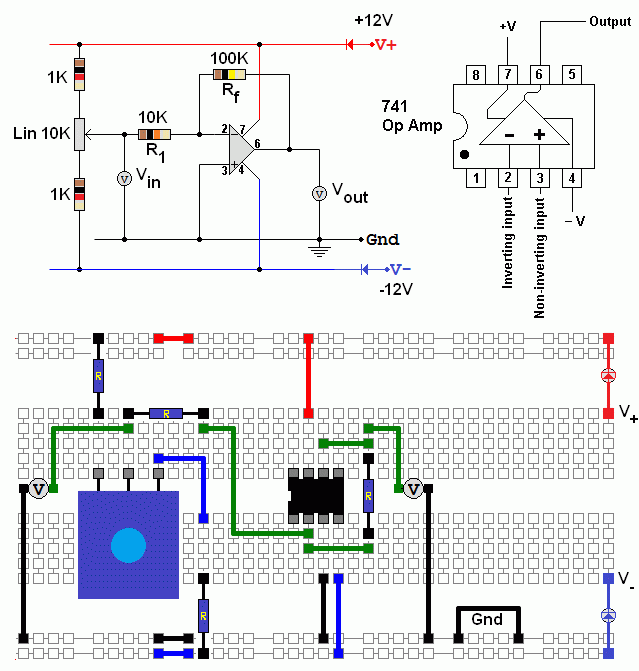Home

rOm
Quest
Glossary

Random
Page
Search
Site
Lush
Sim
Class
Subject
Images

Help
FAQ
Sign
Up
Log
In
 GCSE     Op Amp     Amplifier System     Comparator     Gain     Gain Bandwidth     >Inverting<     Non-Inverting     Power     Summing

# Op Amp Inverting

Site for Eduqas/WJEC - Go to the AQA site.

GCSE    Op AmpsQuestions 0 to 30   -->  View All

Gain   =   - Rf / R1   =   Vout / Vin        Input Impedance   =   R1        The output is 1) bigger and 2) inverted.

Inverting Amplifier in the Lushprojects Simulator• Gain = Vout / Vin
• Gain = - Rf / R1
• Vout / Vin = - Rf / R1
• In the example above, Gain   =   -47 / 10   =   -4.7        It's OK to ignore the "k" because it cancels out.
• Rf and R1 control the GAIN. The formula has a minus sign in it because the amplifier inverts the input signal.
• The input resistance (impedance) of this circuit is equal to R1.
• The output is bigger than the input and inverted.

## Feedback

Feedback is the signal from the Op Amp output, fed back to the input, through Rf. The inverting amplifier uses negative feedback to control the gain.

## The Amplifier

1. Increases the magnitude of the input signal.
2. It inverts the signal. (On an oscilloscope, the waves look upside down).
3. In the oscilloscope trace here, the output (yellow) is four times bigger than the input (red). The gain is MINUS 4.
4. MINUS because the signal is inverted and 4 because it's four times bigger.

## For Maths Experts

Ohm's Law can be used to predict the gain of the Inverting Op Amp.

No current flows into or out of ideal Op Amp inputs. Therefore the current in Rf is equal to the current in R1. Using Ohm's law (V = I R) and since the voltage at P is zero ...

Vin = -I R1          so          I = - Vin / R1

Vout = I Rf          so          I = Vout / Rf

- Vin / R1 = Vout / Rf

Gain = Vout / Vin = - Rf / R1

## Measure the Gain of an Inverting Amplifier

Build this inverting amplifier and measure the gain. The volt meters should be set to the 20 Volt range.

Plot a graph showing Vin on the X axis and Vout on the Y axis. The graph gradient gives you the gain.

Gain = -Rf / R1 = - 100/10 = -10.Subject Name     Level     Topic Name     Question Heading     First Name Last Name Class ID     User ID

 CloseEscapeX Q: qNum of last_q     Q ID: Question ID         Score: num correct/num attempts         Date Done

Question Text

image url

Help Text
Debug

• You can attempt a question as many times as you like.
• If you are logged in, your first attempt, each day, is logged.
• To improve your scores, come back on future days, log in and re-do the questions that caused you problems.
• In the grade book, you can delete your answers for a topic before re-doing the questions. Avoid deleting unless you intend re-doing the questions very soon.